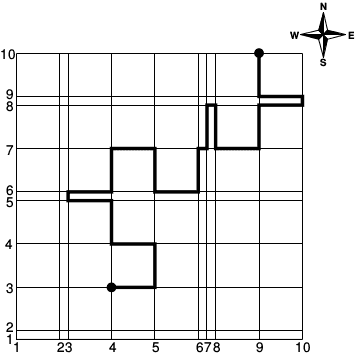### L - Linearville

##### Languages: C, C++, Java, JavaScript, ... (details)The city of Linearville has $N$ parallel two-way streets going in the West-East direction and $N$ parallel two-way streets going in the South-North direction, making up a grid with $(N - 1) \times (N - 1)$ blocks. The distance between two consecutive parallel streets is either $1$ or $5$. The Linearville Transit Authority is conducting an experiment and now requires all cars to always follow a path that alternates direction between W-E and S-N at every crossing, meaning they must turn either left or right when reaching a crossing. The LTA is developing a new navigation app and needs your help to write a program to compute the lengths of shortest alternating paths between many pairs of start and target crossings. The alternating path in the figure, as an example for $N = 10$, is clearly not a shortest alternating path. But beware! Linearville may be huge.

#### Input

The first line contains an integer $N$ $(2 \leq N \leq 10^5)$ representing the number of streets in each direction. For each direction, the streets are identified by distinct integers from $1$ to $N$ starting at the S-W corner of the city. The second line contains $N - 1$ integers $D_1, D_2, ..., D_{N - 1}$ ($D_i \in \{1, 5\}$ for $i = 1, 2, ..., N-1$) indicating the distances between consecutive streets going S-N (that is, $D_i$ is the distance between street $i$ and street $i + 1$). The third line contains $N - 1$ integers $E_1, E_2, ..., E_{N - 1}$ ($E_i \in \{1, 5\}$ for $i = 1, 2, ..., N - 1$) indicating the distances between consecutive streets going W-E (that is, $E_i$ is the distance between street $i$ and street $i + 1$). The fourth line contains an integer $Q$ $(1 \leq Q \leq 10^5)$ representing the number of shortest path queries. Each of the next $Q$ lines describes a query with four integers $A_X, A_Y, B_X$ and $B_Y$ $(1 \leq A_X, A_Y, B_X, B_Y \leq N)$, indicating that the start crossing is $(A_X, A_Y)$ and the target crossing is $(B_X, B_Y)$; the values $A_X$ and $B_X$ are streets going S-N while the values $A_Y$ and $B_Y$ are streets going W-E. There are no repeated queries.

#### Output

Output $Q$ lines, each line with an integer indicating the length of a shortest alternating path for the corresponding query of the input.

#### Sample test(s)

Input
10 5 1 5 5 5 1 1 5 5 1 5 5 5 1 5 5 1 5 3 4 3 9 10 9 2 2 9 5 1 5 10
Output
46 50 49
Input
5 5 1 5 5 5 1 5 5 2 3 1 4 5 5 5 5 5
Output
23 0